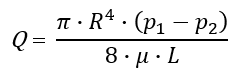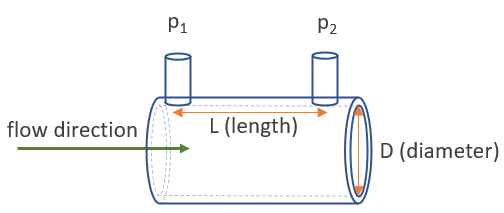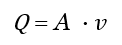# Flow Rate Calculator

Easily calculate the volumetric flow rate of a pipe (a.k.a. discharge rate) given its dimensions and either a pressure difference between its ends or the velocity of the liquid or gas flowing through it. The flow rate calculator can also calculate the mass flow rate of a fluid given its density is known. Input and output support metric and imperial measurement units.

Share calculator:

Embed this tool:
get code

## Using the flow rate calculator

This pipe flow rate calculator calculates the volumetric flow rate (discharge rate) a gas or fluid (liquid) going through a round or rectangular pipe of known dimensions. If the substance is a liquid and its volumetric density is known the calculator will also output the mass flow rate (more information is required to calculate it for gases and it is currently not supported).

In pressure difference mode the calculator requires the input of the pressure before the pipe (or venturi, nozzle, or orifice) as well as at its end, as well as its cross-section, e.g. pressure and diameter for a round pipe. Supported input units include pascals (Pa), bars, atmospheres, pounds per square inch (psi), and more for pressure and kg/m·s, N·s/m2, Pa·s, and cP (centipoise) for dynamic viscosity.

In flow velocity mode one needs to know the flow velocity of the gas or fluid (feet per second, meters per second, km/h, etc. are accepted) in order to calculate the flow rate.

The output is in either imperial or metric units, depending on your selection. Some of the output units include: m3/h, m3/min, m3/s, l/h, l/min, l/s, ft3/h, ft3/min, ft3/s, yd3/h, yd3/min, yd3/s, gallons per hour, gallons per minute. Output units for mass flow rate include: kg/h, kg/mins, kg/s, tonnes/h, lb/h, lb/min, lb/s, tons/h. The output metrics are automatically adjusted for your convenience.

## Flow rate formula

There are two main approaches to calculating the flow rate Q which is equivalent to the difference in volume divided by the difference in time (Δv / Δt). The first one is if we know the pressure difference (pressure drop) between the two points for which we want to estimate the flow. The second one is if we know the fluid velocity. Both are covered below.

### Flow rate formula via pressure difference

Flow rate calculation using pressure is done via the Hagen–Poiseuille equation which describes the pressure drop due to the fluid viscosity . To calculate flow rate from pressure the formula is expressed as such:In the Poiseuille equation (p1 - p2) = Δp is the pressure difference between the ends of the pipe (pressure drop), μ is the dynamic viscosity of the fluid, L and R are the length and radius of the pipe segment in question, and π is the constant Pi ≈ 3.14159 to the fifth significant digit.

There are two main requirements for using the formula above:

• The flow in question should be laminar. This can be established by its Reynolds number. In general the pipe section should not be too wide or too short, otherwise turbulent flows occur.
• The fluid should be incompressible or roughly so. Water is a good example of an incompressible fluid, and so is any hydraulic fluid. Mineral oils, however, are somewhat compressible, so beware of using the formula for such cases.

An example application is if one has manometers measuring the pressure of the fluid or gas at the start an the end of the section of piping that the flow rate is to be calculated for. The graph illustrates a general case where that applies.It should be noted that the Poiseuille formula for calculating a pipe's flow rate through pressure does not work so well for gases where additional information is required for an accurate computation.

### Flow rate formula via fluid velocity

The volumetric flow rate of a stream of liquid or gas is equal to the flow velocity multiplied by its cross-sectional area. Therefore, the formula for flow rate (Q), also known as "discharge rate" expressed in terms of the flow area (A) and its velocity (v) is the so-called discharge equation:The resulting Q is the volumetric flow rate. In the case of a round pipe the cross-sectional area is the inner diameter divided by 2 times π while if it is rectangular the area is the inner width times the inner height. The equation can be transformed in a straightforward way to allow for solving for the cross-section area or velocity.

### Mass flow rate formula

The mass flow rate ṁ is the flow of mass m through a surface per unit time t, therefore the formula for mass flow rate, given the volumetric flow rate, is ṁ = Q * ρ where ρ (Greek lower-case letter rho) is the volumetric density of the substance. This equation is applicable to liquids whereas for gaseous substances some additional information is required to perform the calculations.

## Calculation examples

Example 1: A round pipe has a diameter of 25 mm and water is running through it with a velocity of 10 m/s. What is the flow rate of the water? First, we calculate the cross-section area to be (25/2)^2 · 3.1416 ~= 490.875 mm2 via the area of a circle formula. We can convert this to m2 by dividing by 1,000,000 for more convenient results, getting 0.000490875 m2. Using the flow rate equation above we replace the values for A and v and get Q = 0.000490875 m2 · 10 m/s) = 0.00490875 m3/s. To convert this to m3/h we need to multiply by 3,600 to get a discharge rate of 17.6715 m3 per hour.

If we further know that the density of water is 1000 kg/m3 we can calculate the mass flow rate to be 17.6715 m3/h · 1000 kg/m3 = 17671.5 kg/h (= 17.6715 tonnes per hour, m3 cancels out).

Example 2: A rectangular pipe has a height of 2cm and width of 4cm and a gas running through it at a speed of 15 m/s. What is the discharge rate of this pipe? First, we find the cross-section area via the formula for the area of a rectangle which is simply 2 · 4 = 8 cm2 or 0.0008 m2. To find the flow rate Q, we multiply 0.0008 by 15 to get 0.012 cubic meters per second. To get litres per second we simply need to multiply by 1,000 to get 12 l/s. If we wanted to get liters per hour, we can further multiply by 3600 to get 43,200 litres per hour.

Our calculator is especially useful if the input units for the calculation are different from the desired output units, in which case it will perform these unit conversions for you.